• 哈夫曼树
千次阅读
2017-07-26 17:26:28

•哈夫曼树，第一行输入一个数n，表示叶结点的个数。需要用这些叶结点生成哈夫曼树，根据哈夫曼树的概念，这些结点有权值，即weight，题目需要输出所有结点的值与权值的乘积之和。
输入：



•输入有多组数据。
•每组第一行输入一个数n，接着输入n个叶节点（叶节点权值不超过100，2<=n<=1000）。
输出：
•输出权值。
样例输入：
•5
•1 2 2 5 9
样例输出：
•37

算法一：

nclude<iostream>
#include<stack>
using namespace std;
int result;
//哈夫曼树的权值就是除了所有叶子  

//的节点的权值的和
int main(){
int n,i,sum,num;
while(scanf("%d",&n)!=EOF){
memset(result,0,n);
for(i=0;i<n;i++)
scanf("%d",&result[i]);
//进行排序,从小到大
sort(result,result+n);
i=1;
sum=0;
while(i<n)
{
//每次都要进行重新排序，因为生成了新的节点
sort(result+i-1,result+n);
//计算父亲
num = result[i-1]+result[i];
sum+=num;
//将新的节点赋值
result[i]=num;
i++;
}
printf("%d\n",sum);
}
return 0;
}  
算法二：

#include <string.h>
#include <algorithm>
#include<iostream>
#include<stack>
#define maxvalue 0x7fffffff//这个是int的最大值
using namespace std;
//创建节点的结构体
struct huffman{
int weight;
int parent,lchild,rchild;
}list;
int main()
{
int n,m;
int i,j;
int ans;
int x1,x2;//用来存放树生成过程中的最小和次小的角标
int m1,m2;//用来存放树生成过程中的最小和次小的值
while(scanf("%d",&n)!=EOF)
{
m=2*n-1; //生成2n-1个节点
for(i=0;i<m;i++)
list[i].parent=list[i].lchild=list[i].rchild=-1;
for(i=0;i<n;i++)
scanf("%d",&list[i].weight);
ans=0;
for(i=0;i<n-1;i++)
{
x1=x2=0;
m1=m2=maxvalue;
for(j=0;j<n+i;j++)
{
//用来判断新的节点是否小于最小且没有双亲
//如果小于最小的话就把当前的数和角标给x1和m1
//并且在x1和m1中存入当前最小的角标和值
if(list[j].weight<m1&&list[j].parent==-1)
{
x2=x1;
m2=m1;
x1=j;
m1=list[j].weight;
}
//用来判断是不是小于次小，如果小于的话就替换次小
else if(list[j].weight<m2&&list[j].parent==-1)
{
x2=j;
m2=list[j].weight;
}
}
list[x1].parent=n+i;
list[x2].parent=n+i;
list[n+i].lchild=x1;
list[n+i].rchild=x2;
list[n+i].weight=list[x1].weight+list[x2].weight;
ans+=list[n+i].weight;
}
printf("%d\n",ans);
}
return 0;
}

算法三：这个是针对于此类型的题比较完整的算法，可我现在还不太理解，对指针还不熟悉，可能以后回过来看会理解。
/*示例
****哈夫曼编码****
请输入结点个数：8
输入这8个元素的权值（均为整形）：
1:27
2:4
3:87
4:21
5:2
6:21
7:1
8:25

*/
#include <stdio.h>
#include <stdlib.h>
#include <string.h>

typedef struct
{
unsigned int weight;  //用来存储各个结点的权值
unsigned int parent,LChild,RChild;  //指向双亲、孩子结点的指针
} HTNode, *HuffmanTree;  //动态分配数组，存储哈夫曼树

typedef char *HuffmanCode;  //动态分配数组，存储哈夫曼树

///选择两个parent为0,且weight最小的结点s1和s2
void Select(HuffmanTree *ht,int n,int *s1,int *s2)
{
int i,min;
for(i=1; i<=n; i++)
{
if((*ht)[i].parent==0)
{
min=i;
break;
}
}
for(i=1; i<=n; i++)
{
if((*ht)[i].parent==0)
{
if((*ht)[i].weight<(*ht)[min].weight)
min=i;
}
}
*s1=min;

for(i=1; i<=n; i++)
{
if((*ht)[i].parent==0 && i!=(*s1))
{
min=i;
break;
}
}
for(i=1; i<=n; i++)
{
if((*ht)[i].parent==0 && i!=(*s1))
{
if((*ht)[i].weight<(*ht)[min].weight)
min=i;
}
}
*s2=min;
}

///构造哈夫曼树ht,w存放已知n个权值
void CrtHuffmanTree(HuffmanTree *ht,int *w,int n)
{
int m,i,s1,s2;
m=2*n-1;    //总共的结点数
*ht=(HuffmanTree)malloc((m+1)*sizeof(HTNode));
for(i=1; i<=n; i++)  //1-n号存放叶子结点，初始化
{
(*ht)[i].weight=w[i];
(*ht)[i].LChild=0;
(*ht)[i].parent=0;
(*ht)[i].RChild=0;
}
for(i=n+1; i<=m; i++)   //非叶子结点的初始化
{
(*ht)[i].weight=0;
(*ht)[i].LChild=0;
(*ht)[i].parent=0;
(*ht)[i].RChild=0;
}

printf("\n?哈夫曼树为: \n");

for(i=n+1; i<=m; i++)   //创建非叶子结点，建哈夫曼树
{ /*在(*ht)~(*ht)[i-1]的范围内选择两个parent为0且weight最小的结点，其序号分别赋值给s1、s2*/
Select(ht,i-1,&s1,&s2);
(*ht)[s1].parent=i;
(*ht)[s2].parent=i;
(*ht)[i].LChild=s1;
(*ht)[i].RChild=s2;
(*ht)[i].weight=(*ht)[s1].weight+(*ht)[s2].weight;
printf("%d (%d, %d)\n",(*ht)[i].weight,(*ht)[s1].weight,(*ht)[s2].weight);
}
printf("\n");
}

//从叶子结点到根，逆向求每个叶子结点对应的哈夫曼编码
void CrtHuffmanCode(HuffmanTree *ht, HuffmanCode *hc, int n)
{
char *cd;   //定义的存放编码的空间
int a;
int i,start,p,w=0;
unsigned int c;
hc=(HuffmanCode *)malloc((n+1)*sizeof(char *));  //分配n个编码的头指针
cd=(char *)malloc(n*sizeof(char));  //分配求当前编码的工作空间
cd[n-1]='\0';  //从右向左逐位存放编码，首先存放编码结束符

for(i=1; i<=n; i++)  //求n个叶子结点对应的哈夫曼编码
{
a[i]=0;
start=n-1;  //起始指针位置在最右边
for(c=i,p=(*ht)[i].parent; p!=0; c=p,p=(*ht)[p].parent)  //从叶子到根结点求编码
{

if( (*ht)[p].LChild==c)
{
cd[--start]='1';  //左分支标1
a[i]++;
}
else
{
cd[--start]='0';  //右分支标0
a[i]++;
}
}
hc[i]=(char *)malloc((n-start)*sizeof(char));  //为第i个编码分配空间
strcpy(hc[i],&cd[start]);    //将cd复制编码到hc
}
free(cd);
for(i=1; i<=n; i++)
printf(" 权值为%d的哈夫曼编码为：%s\n",(*ht)[i].weight,hc[i]);
for(i=1; i<=n; i++)
w+=(*ht)[i].weight*a[i];
printf(" 带权路径为：%d\n",w);

}

int main()
{
HuffmanTree HT;
HuffmanCode HC;
int *w,i,n,wei;

printf("**哈夫曼编码**\n" );
printf("请输入结点个数：" );
scanf("%d",&n);
w=(int *)malloc((n+1)*sizeof(int));
printf("\n输入这%d个元素的权值:\n",n);

for(i=1; i<=n; i++)
{
printf("%d: ",i);
fflush(stdin);//清空输入缓冲区；
scanf("%d",&wei);
w[i]=wei;
}
CrtHuffmanTree(&HT,w,n);
CrtHuffmanCode(&HT,&HC,n);

system("pause");
return 0;
}

更多相关内容
•数据结构
• 1． 输出存放哈夫曼树的数组HT初态和终态； 2． 输出每个字符的哈夫曼编码； 3． 输入由上述若干字符组成的字符串，对电文进行编码并输出； 4． （选作）输入电文的哈夫曼编码，进行译码并输出。
• ## 哈夫曼树（HuffmanTree）详解

千次阅读 多人点赞 2018-11-22 22:16:20
目录 1.哈夫曼算法 2.举例说明，感性认识...7. 打印哈夫曼树和哈夫曼编码 8.完整代码 9.运行截图 1.哈夫曼算法 1.根据给定的n个权值{w1,w2,...,wn}构造n棵二叉树的集合F={T1,T2,...,Tn}，其中每棵二叉树Ti...

目录

1.哈夫曼算法

2.举例说明，感性认识哈夫曼算法，根据上面的4步得出下表

3.结点结构体类型的定义

4.select函数的编写

5.哈夫曼树的创建及编码的创建

6.解码

7. 打印哈夫曼树和哈夫曼编码

8.完整代码

9.运行截图

### 1.哈夫曼算法

• 1.根据给定的n个权值{w1,w2,...,wn}构造n棵二叉树的集合F={T1,T2,...,Tn}，其中每棵二叉树Ti中只有一个带权为wi的根结点，其左右子树均空。
• 2.F中选取两棵根结点的权值最小的树作为左右子树构造一棵新的二叉树，且置新的二叉树的根结点的权值为其左、右子树上根结点的权值之和。
• 3.F中删除这两棵树，同时将新得到的二叉树加入F中。
• 4.重复23，直到F中只含一棵树为止。这棵树便是最优二叉树。
• 最优二叉树是一类带权路径长度最短的树。

### 2.举例说明，感性认识哈夫曼算法，根据上面的4步得出下表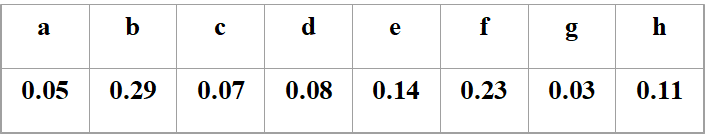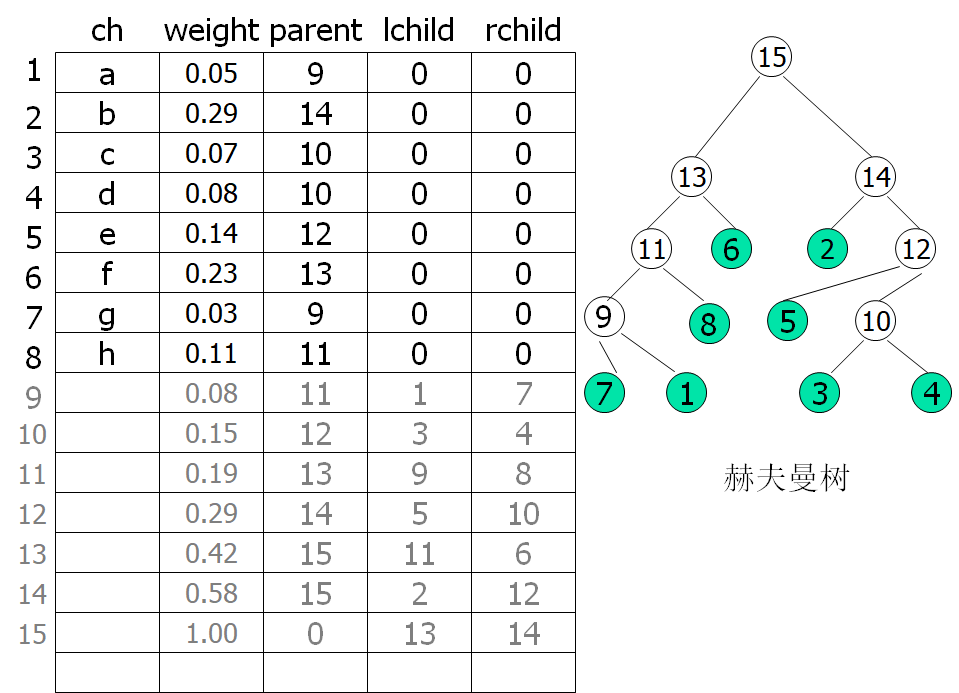### 3.结点结构体类型的定义typedef struct {
char ch;
int weight;
int parent,lchild,rchild;
}HTNode,*Huffmantree;

### 4.select函数的编写

/用s1和s2返回前end个结点中最小权重和次小权重的序号
思路：将未被安排的结点的序号赋给s1,s2，从下一位开始，
依次更新s1和s2的值，最后将这两个权重较小的序号给s1,较大的给s2.

Status select(Huffmantree HT,int end,int &s1,int &s2)
{
for(i=1,count=1;i<=end;i++)
{

//结点成员parent值为0表明该结点还未被安排
//将第一个，第二个未被安排的结点序号赋给m,n
if(HT[i].parent ==0)
{
if(count==1)
m=i;
if(count==2)
n=i;
count++;
}
if(count>2)
break;
}

//令m为结点较小权值的序号，令n为较大的序号
if(HT[m].weight>HT[n].weight)
{
tmp=n;
n=m;
m=tmp;
}

//i从下一个开始，一直扫描到end
i=(m>n?m:n)+1;

while(i<=end)
{

//同样寻找未被安排的结点
if(HT[i].parent==0)
{
//i的权重比m的小,则用m替n, i替m
if(HT[i].weight<HT[m].weight)
{
n=m;
m=i;
}

//i的权重介于m和n之间，则用i替n
//还剩一种情况，当i比n大时，不做任何改变
//故在此分为了两类
else
{
if(HT[i].weight>=HT[m].weight&&
HT[i].weight<HT[n].weight)
n=i;
}
}
i++;
}

//用s1返回最小权重序号
//用s2返回次小权重序号
s1=HT[m].weight<=HT[n].weight?m:n;
s2=HT[m].weight>HT[n].weight?m:n;
return ok;
}


### 5.哈夫曼树的创建及编码的创建

• //w[]存放n个字符的权值，str存放n个字符名ch，构造赫夫曼树HT，并求出n个字符的编码
• int HuffmanCoding(Huffmantree &HT,char** &HC, int *w, int n,char *str)

主要思路：

n个叶子结点对应2n+1个总结点，对序号从1到n的结点

HT[i].weight = w[i-1];
HT[i].parent = 0;
HT[i].lchild = HT[i].rchild = 0;
HT[i].ch=str[i-1];

对序号从n+1到2n+1的结点：

HT[i].ch='\0';   //要是不赋为空的话为给出随机字符
HT[i].parent = 0;
HT[i].lchild = HT[i].rchild = 0;

• //构造赫夫曼树,m=2*n-1
• //从HT[1..i-1]中选择parent为0且weight最小的两个结点，其序号为s1和s2

select(HT,i-1,s1,s2);
HT[s1].parent = i;     HT[s2].parent = i;
HT[i].lchild = s1;       HT[i].rchild = s2;
HT[i].weight = HT[s1].weight + HT[s2].weight;

• //从叶子到根逆向求每个字符的赫夫曼编码

for(int c=i,f=HT[i].parent;f!=0;c=f,f=HT[f].parent)
{
if (HT[f].lchild == c)   cd[--start]='0';
else cd[--start]='1';
}
HC[i] = (char*)malloc((n-start)*sizeof(char));
strcpy(HC[i],&cd[start]);

### 6.解码

• //将二进制编码翻译回信息原文，m是树根的编号
• int  Decoding(Huffmantree HT,int m,char *buff)

if ((*buff)=='0')
p = HT[p].lchild; //进入左分支
else
p = HT[p].rchild; //进入右分支
buff++;
if (!HT[p].lchild && !HT[p].rchild) {
//进入叶子结点
printf("%c",HT[p].ch);
p = m; //重新从树根出发进行译码

### 7. 打印哈夫曼树和哈夫曼编码

• //以表格的方式打印哈夫曼树
• void ShowHuffmanTree(Huffmantree HT,int n)
• //以表格形式打印哈夫曼编码
• void ShowHuffmanCode(Huffmantree HT,char** HC,int n)
• 主要运用printf函数格式输出。

### 8.完整代码

#include<stdio.h>
#include<stdlib.h>
#include<string.h>

#define ok 1

//赫夫曼树的存储结构
typedef struct {
char ch;
int weight;
int parent,lchild,rchild;
}HTNode,*Huffmantree;
typedef int Status;

//用s1和s2返回前end个结点中最小权重和次小权重的序号
Status select(Huffmantree HT,int end,int &s1,int &s2)
{
int i,count;
int m,n,tmp;
if(end<2)
return 0;
for(i=1,count=1;i<=end;i++)
{
if(HT[i].parent ==0)
{
if(count==1)
m=i;
if(count==2)
n=i;
count++;
}
if(count>2)
break;
}
if(HT[m].weight>HT[n].weight)
{
tmp=n;
n=m;
m=tmp;
}
i=(m>n?m:n)+1;
while(i<=end)
{
if(HT[i].parent==0)
{
if(HT[i].weight<HT[m].weight)
{
n=m;
m=i;
}
else
{
if(HT[i].weight>=HT[m].weight&&HT[i].weight<HT[n].weight)
n=i;
}
}
i++;
}
s1=HT[m].weight<=HT[n].weight?m:n;
s2=HT[m].weight>HT[n].weight?m:n;
return ok;
}

//w[]存放n个字符的权值，str存放n个字符名ch，构造赫夫曼树HT，并求出n个字符的编码
int HuffmanCoding(Huffmantree &HT,char** &HC, int *w, int n,char *str)
{
int i,m;
if (n<=1)  return 0;
m = 2*n-1;
HT =(Huffmantree)malloc((m+1)*sizeof(HTNode));
for(i=1; i<=n; i++)
{
HT[i].weight = w[i-1];
HT[i].parent = 0;
HT[i].lchild = HT[i].rchild = 0;
HT[i].ch=str[i-1];
}
for(; i<=m; ++i)//m=2*n-1
{
HT[i].ch='\0';
HT[i].parent = 0;
HT[i].lchild = HT[i].rchild = 0;
}
for(i=n+1; i<=m; i++)
{//构造赫夫曼树,m=2*n-1
//从HT[1..i-1]中选择parent为0且weight最小的两个结点，其序号为s1和s2
int s1, s2;
select(HT,i-1,s1,s2);
HT[s1].parent = i;     HT[s2].parent = i;
HT[i].lchild = s1;       HT[i].rchild = s2;
HT[i].weight = HT[s1].weight + HT[s2].weight;
}
//从叶子到根逆向求每个字符的赫夫曼编码
HC = (char **)malloc((n+1)*sizeof(char*));
char *cd;
cd = (char *)malloc(n*sizeof(char));
cd[n-1] = '\0';
for(i=1; i<=n; i++)
{
int start = n-1;
for(int c=i,f=HT[i].parent;f!=0;c=f,f=HT[f].parent)
{
if (HT[f].lchild == c)   cd[--start]='0';
else cd[--start]='1';
}
HC[i] = (char*)malloc((n-start)*sizeof(char));
strcpy(HC[i],&cd[start]);
//printf("%c的哈夫曼编码是%s\n",HT[i].ch,HC[i]);
}
free(cd);

return ok;
}//HuffmanCoding

//将二进制编码翻译回信息原文，m是树根的编号
int  Decoding(Huffmantree HT,int m,char *buff)
{
int p = m;
while (*buff != '\0' && p != 0) {
if ((*buff)=='0')
p = HT[p].lchild; //进入左分支
else
p = HT[p].rchild; //进入右分支
buff++;
if (!HT[p].lchild && !HT[p].rchild) {
//进入叶子结点
printf("%c",HT[p].ch);
p = m; //重新从树根出发进行译码
}//if
}//while
return ok;
}//Decoding

void ShowHuffmanTree(Huffmantree HT,int n)
{
int i;
printf("┍┉┉┉┉┉┉┉┉┱┉┉┉┉┉┉┉┉┱┉┉┉┉┉┉┉┉┲┉┉┉┉┉┉┉┉┲┉┉┉┉┉┉┉┉┲┉┉┉┉┉┉┉┉┒\n");
printf("┋   ch   ┋ order  ┋ weight ┋ parent ┋ lchild ┋ rchild ┋\n");
for(i=1;i<=n;i++)
printf("┋   %c    ┋   %2d   ┋   %3d  ┋   %2d   ┋   %2d   ┋   %2d   ┋\n",
HT[i].ch,i,HT[i].weight,HT[i].parent,HT[i].lchild,HT[i].rchild);
printf("┖┉┉┉┉┉┉┉┉┺┉┉┉┉┉┉┉┉┺┉┉┉┉┉┉┉┉┺┉┉┉┉┉┉┉┉┺┉┉┉┉┉┉┉┉┺┉┉┉┉┉┉┉┉┚\n");
}

void ShowHuffmanCode(Huffmantree HT,char** HC,int n)
{
int i;
printf("┍┉┉┉┉┉┉┉┉┱┉┉┉┉┉┉┉┉┲┉┉┉┉┉┉┉┉┲┉┉┉┉┉┉┉┉┲┉┉┉┉┉┉┉┉┒\n");
printf("┋   ch   ┋ order  ┋ weight ┋        ┋  Code  ┋\n");
for(i=1;i<=n;i++)
printf("┋   %c    ┋   %2d   ┋   %2d   ┋  ---->  %-8s┋\n",
HT[i].ch,i,HT[i].weight,HC[i]);
printf("┖┉┉┉┉┉┉┉┉┺┉┉┉┉┉┉┉┉┺┉┉┉┉┉┉┉┉┺┉┉┉┉┉┉┉┉┺┉┉┉┉┉┉┉┉┚\n");
}

int main()
{
int n,m;
printf("请输入叶子结点的个数：");
scanf("%d",&n);
int w[n];
printf("\n请依次输入各结点的权值：\n");
for(int i=0;i<n;i++)
scanf("%d",&w[i]);
char str[n];
printf("\n给叶子结点起个名字：\n");
scanf("%s",str);
Huffmantree HT;char** HC;
printf("\n哈夫曼树构建中...\n\n");
HuffmanCoding( HT, HC, w,  n, str);
printf("打印哈夫曼树：\n");
ShowHuffmanTree( HT,2*n-1);
printf("\n打印叶子结点的哈夫曼编码：\n");
ShowHuffmanCode( HT, HC, n);

printf("\n执行解码操作，请输入一串哈夫曼编码：\n");
char buff;
scanf("%s",buff);
printf("解码为：\n");
Decoding( HT, 2*n-1 ,buff);
printf("\n");
system("pause");
return 0;
}

/* test data
8
5 29 7 8 14 23 3 11
abcdefgh
a的哈夫曼编码是0001
b的哈夫曼编码是10
c的哈夫曼编码是1110
d的哈夫曼编码是1111
e的哈夫曼编码是110
f的哈夫曼编码是01
g的哈夫曼编码是0000
h的哈夫曼编码是001
*/

### 9.运行截图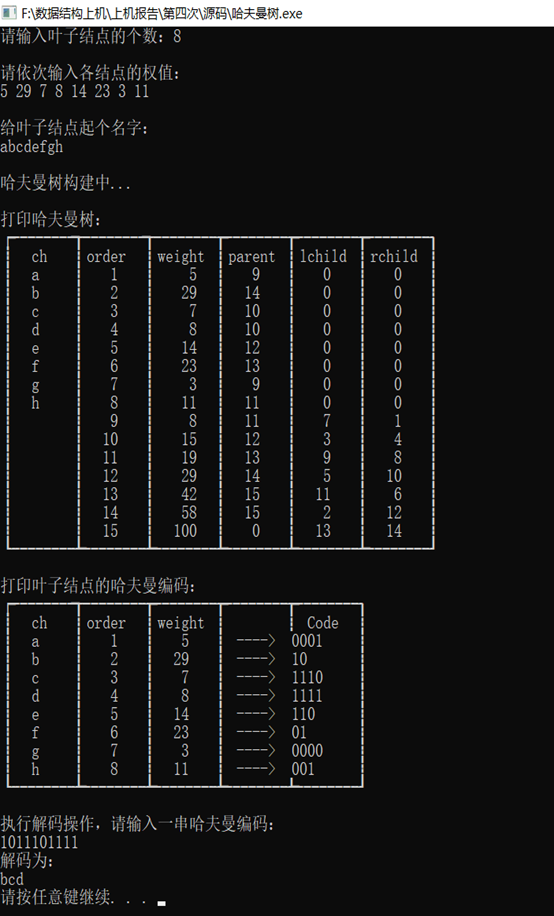展开全文数据结构
• 从键盘输入字符集（字母a～z，空格）共27个字符，以及出现的频率，将字符出现的频率作为结点的权值，建立哈夫曼树，并输出数组ht[]的初态和终态。 对各个字符进行哈夫曼编码，打印输出字符及对应的哈夫曼编码。 ...
• 一、一些基本概念 1、路径：从树中一个结点到另一个结点之间的分支构成这两个结点之间的路径。...7、哈夫曼树：假设有m个权值{w1,w2,…,wm}，可以构造一棵含n个叶子结点的二叉树，每个叶子结点的权为

### 一、一些基本概念

1、路径：从树中一个结点到另一个结点之间的分支构成这两个结点之间的路径。
2、路径长度：路径上的分支数目称作路径长度。
3、树的路径长度：从树根到每一结点的路径长度之和。
4、权：赋予某个实体的一个量，是对实体的某个或某些属性的数值化描述。
5、结点的带权路径长度：从该结点到树根之间的路径长度与结点上权的乘积。
6、树的带权路径长度：树中所有叶子结点的带权路径长度之和，通常记为: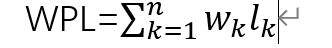7、哈夫曼树：假设有m个权值{w1,w2,…,wm}，可以构造一棵含n个叶子结点的二叉树，每个叶子结点的权为wi，则其中带权路径长度WPL最小的二叉树称为最优二叉树或哈夫曼树。
注意：
（1）完全二叉树不一定是哈夫曼树；
（2）权值越大的结点越靠近根结点；
（3）哈夫曼树不唯一，但其树的带权路径长度一定相等；

## 二、构造哈夫曼树

哈夫曼树结点结构：哈夫曼树的存储表示

typedef struct{
int weight;  //结点的权值
int parent,lchild,rchild;  //结点的双亲、左孩子、右孩子的下标
}HTNode,*HuffmanTree;  //动态分配数组存储哈夫曼树


哈夫曼树结点个数：例题：已知w=(5,29,7,8,14,23,3,11),生成一棵哈夫曼树，计算树的带权路径长度。并给出其构造过程中存储结构HT的初始状态和终结状态。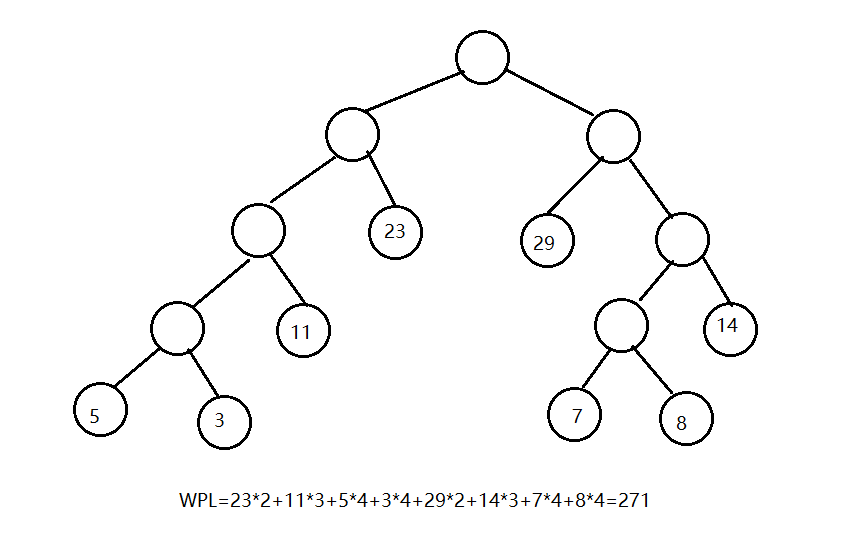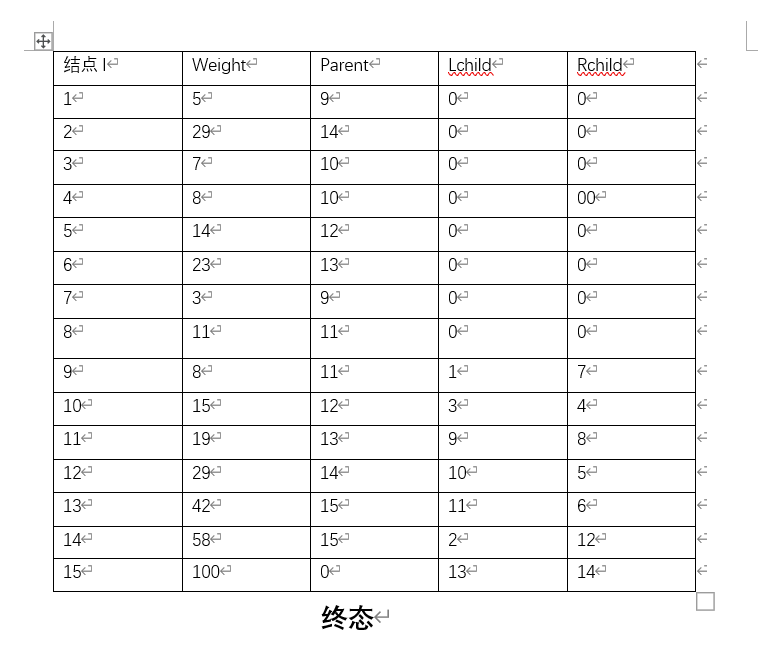【算法描述】

void CreateHT(HuffmanTree &HT,int n)
{
if(n<=1)return ;
m=2*n-1;
HT=new HTNode[m+1];
for(i=1;i<=m;++i)    //将1-m号单元的父结点、左孩子、右孩子的下标都初始化为0
{
HT[i].parent=0;
HT[i}.lchild=0;
HT[i}.rchild=0;
}
for(i=1;i<=n;++i)  //输入前n个结点的权值
{
cin>>HT[i].weight;
}
for(i=n+1;i<=m;++i)
{ //通过n-1次的选择、删除、合并来创建哈夫曼树
Select(HT,i-1,s1,s2);
//在HT[k](1<=k<=i-1)中选择两个其双亲域为0且权最小的结点，并返回他们在HT中的序号s1和s2
HT[s1}.parent=i;
HT[s2}.parent=i;
//得到新结点i，从森林中删除s1,s2，将s1和s2的双亲域由0改为1
HT[i].lchild=s1;   //s1作为i的左结点
HT[i}.rchild=s2;  //s2作为i的右结点
HT[i].weight=HT[s1].weight+HT[s2].weight;  //i的权值为左右孩子权值之和
}


## 三、哈夫曼编码

将树的左分支标记为0，右分支标记为1；（左0右1）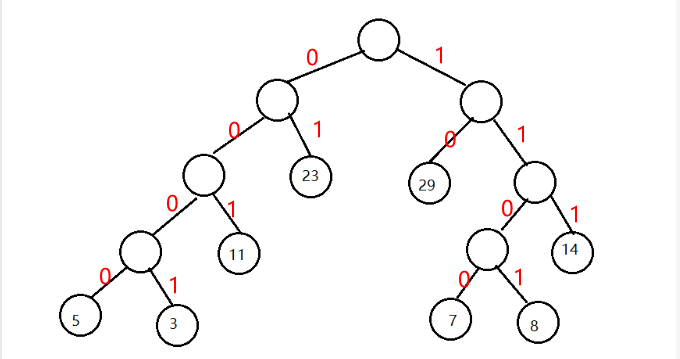哈夫曼编码
50 0 0 0
30 0 0 1
110 0 1
230 1
291 0
141 1 1
71 1 0 0
81 1 0 1

## 四、哈夫曼树的创建

要求：
1、从键盘输入n, 以及n个字符的概率。
例如：已知某系统在通信联络中只可能出现n种字符，其概率分别为 0.05, 0.29, 0.07, 0.08, 0.14, 0.23, 0.03, 0.11，试设计哈夫曼编码创建哈夫曼树。
2、用顺序存储。

#include<iostream>
using namespace std;
//哈夫曼树的存储结构
typedef struct {
int weight;  //结点的权重
int parent, lchild, rchild;  //结点的双亲、左孩子、右孩子的下标
}HTNode,*HuffmanTree;
//封装两个最小结点
typedef struct {
int s1;
int s2;
}MIN;
//选择双亲为0且结点权值最小的两个结点
MIN Select(HuffmanTree HT, int n)
{
int min, secmin,s1,s2;
min = 10000;
secmin = 10000;
MIN code;
s1 = 1; s2 = 1;
for (int i = 1; i <= n; i++)
{
if (HT[i].parent == 0 && (HT[i].weight<min))
{
min = HT[i].weight;
s1 = i;
}
}
for (int i = 1; i <= n; i++)
{
if (HT[i].parent == 0 && (HT[i].weight<secmin) && (i != s1))
{
secmin = HT[i].weight;
s2 = i;
}
}
code.s1 = s1;
code.s2 = s2;
return code;

}
//创造哈夫曼树
void CreateHuffmanTree(HuffmanTree &HT, int num)
{

int m;
m = 2 * num - 1;
HT = new HTNode[m + 1];
for (int i = 1; i <= m; i++)
{
HT[i].parent = 0;
HT[i].lchild = 0;
HT[i].rchild = 0;
}
cout << "请输入每个叶子结点的权值：" << endl;
for (int i = 1; i <= num; i++)
{
cin >> HT[i].weight;
}

for (int i = num + 1; i <= m; i++)
{
MIN min;
min=Select(HT,i-1);
HT[min.s1].parent = i;
HT[min.s2].parent = i;
HT[i].lchild = min.s1;
HT[i].rchild = min.s2;
HT[i].weight = HT[min.s1].weight + HT[min.s2].weight;
}
//输出哈夫曼树存储结构的末态
for (int i = 1; i <= m; i++)
{
cout << "结点序号 " << i << " 权重 " << HT[i].weight << " parent " << HT[i].parent << " lchild " << HT[i].lchild << " rchild " << HT[i].rchild << endl;
}

}

int main()
{
cout << "开始创建哈夫曼树" << endl;
int num;  //结点的个数
cout << "请输入哈夫曼树叶子结点的个数：";
cin >> num;
//创造哈夫曼树
HuffmanTree HT;
CreateHuffmanTree(HT, num);
return 0;
}

展开全文数据结构
• ## 【笔记】哈夫曼树

千次阅读 2017-11-01 00:34:54
哈夫曼树的概念 哈夫曼树的构造算法 哈夫曼编码 哈夫曼编码算法的实现

哈夫曼树又称最优二叉树。它是一种带权路径长度最短的树，应用非常广泛。

# 1.哈夫曼树的概念

• 扩充二叉树

对每棵二叉树进行扩充：每当在原来的二叉树中出现空子树时，就加上一个特殊的结点。显然，每个内节点都有两个儿子，而每个方结点都没有儿子，如果二叉树有n个内结点和S个外结点，则S=n+1，即外结点的个数比内结点的个数多1.

设已按此法将第一颗二叉树加以扩充，树的外路长（用E表示）定义为从根结点到外结点的路长之和，而內路长（用I表示）定义为从根结点到每个内结点的路长之和。他们总是满足E=I+2n

• 路径和路径长度

路径是指在树中一个结点到另一个结点所走过的路程。路径长度是一个结点到另一个结点之间的分支数目。树的路径长度是指从树根到每个结点的路径长度的和。

• 树的带权路径长度

结点的带权路径长度为从该结点到树根之间的路径长度与即诶但上权的乘积。树的带权路径长度为树中所有叶子结点的带权路径长度之和，通常记作 WPL=ni=1wi×li $WPL=\sum^n_{i=1}w_i \times l_i$，其中，n是树中叶子结点的个数， wi $w_i$是第i个叶子结点的权值， li $l_i$第i个叶子结点路径长度。加权路长的应用之一是把二叉树看成一个判断过程：从根开始做某种测试，根据测试的结果选择两个分支之一，而在分支中可以做进一步的测试等。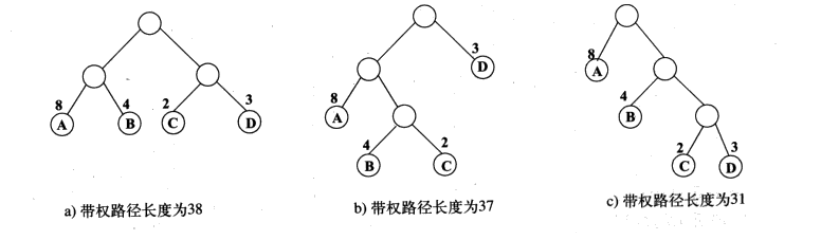注意：加权路长最小者并非一定是完全平衡的二叉树。

# 2.哈夫曼树的构造算法

哈夫曼树就是带权路径长度最小的树，权值最小的结点原理根结点，权值越大的结点越靠近根结点。
哈夫曼树的构造算法：

1. 由给定的n个权值{ w1,w2,,wn $w_1,w_2,…,w_n$}构成n棵只有根结点的二叉树集合 F=T1,T2,,Tn $F={T_1,T_2,…,T_n}$，其中每棵二叉树 Ti $T_i$中只有一个带权为 wi $w_i$的根结点，其左右子树均为空。
2. 在二叉树集合 F <script type="math/tex" id="MathJax-Element-16">F</script>中选区两棵根结点的权值最小的和次小的的树作为左、右子树构造一棵新的二叉树，新二叉树的根结点的权重为这两棵子树根结点的权重之和。
3. 在二叉树集合F中删除这两棵二叉树，并将新得到的二叉树加入到集合F中。
4. 重复步骤2和3，指导结合F中只剩下一棵二叉树为止。这棵树就是最优二叉树——哈夫曼树。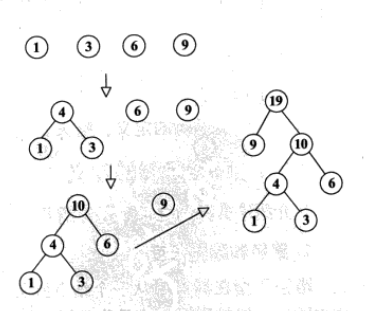# 3.哈夫曼编码

在电报的传输过程中，需将传送的文字转换成二进制的字符组成的字符串。在传送电文时，希望电文的长度尽可能短。如果按照每个字符进行长度不等的编码，将出现频率高的字符采用尽可能短的编码，则电文的代码长度就会减少。用一个二进制数字串对每个字符进行编码，使任意一个字符的编码不会是任何其他字符编码的前缀。通常把编码的这种特性叫做前缀性，前缀性使两个字符编码之间不需要加分隔符。可以按下述方法对二进制数字进行译码：反复删去该串的前缀，这些前缀就是一些字符的编码。因此所设计的长度不等的编码必须满足任意一个字符的编码都不是另一个字符的前缀的要求，这样的编码称为前缀编码

可以将前缀编码看成二叉树中的路径。每个结点的左分支附以一个0，而结点的右分支附以一个1，将字符作为叶结点的标号。从根结点到叶结点的路径上遇到0或1构成的序列就是相应的字符的编码。因此任意一种前缀编码都可以用一棵二叉树来表示。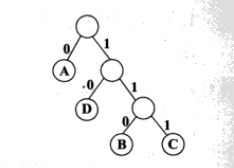哈夫曼编码算法的基本思想：从给定的字符集中选择出现概率最小的两个字符a、b；用一个字符（如x）代替a和b，而x的概率对应于a和b的概率之和。然后，对新的、字符个数较少的字符集（去掉a、b而加上x）递归地求最佳前缀编码。原来的字符集中字符的编码可以这样得到：a的编码是在x编码后附以0，而b的编码是在x的编码后附以1。
每棵树中叶结点的标号是要编码的字符，其跟记载该树所有叶结点字符所对应的概率之和，此和数称为该树的权。起初，每个字符本身是一棵树；当算法那结束时，形成唯一的一棵树，所有的字符都在它的叶结点上。从根结点到叶结点的路径上的0、1序列就表示该叶结点标号的编码。对于给定的字符集和出现的概率，哈夫曼树所表示的字符的编码的平均长度最小。

# 4.哈夫曼编码算法的实现

假设一个字符序列为｛a,b,c,d｝，对应的权重为{2,3,6,8}。构造一棵哈夫曼树，然后输出相应的哈夫曼编码。

• 哈夫曼树的类型定义
typedef struct
{
unsigned int weight;
unsigned int parent,lchild,rchild;
}HTNode,*HuffmanTree;
typedef char **HuffmanCode; /*存放哈夫曼编码*/

HuffmanCode为一个二级指针，相当于二维数组，用来存放每一个叶子结点的哈夫曼编码。起初时，将每一个叶子结点的双亲结点域、左孩子域和右孩子域都初始化为0。若有n个叶子结点，则非叶子结点有n-1个，所以总共结点数目是2n-1个。同时也要将剩下的n-1个双亲结点域初始化为0，这主要是为了查找权值最小的结点方便。

• 创建哈夫曼树并构造哈夫曼编码

一次选择两个权值最小的结点s1和s2分别作为左子树结点和右子树结点，并为其双亲结点赋予一个地址，双亲结点的权值为s1和s2的权值之和。修改它们的parent域，使它们指向同一个双亲结点，双亲结点的左子树为权值最小的结点，右子树为权值次小的结点。重复执行这种操作n-1次，即求出n-1个非叶子结点的权值。这样就构造出了一棵哈夫曼树。

求哈夫曼编码的方式有两种，即从根结点开始到叶子结点正向求哈夫曼编码和从叶子结点到根结点你想求哈夫曼编码，这里给出从根结点到叶子结点求哈夫曼编码的算法：
从编号为2n-1的结点开始，即根结点开始，依次通过判断左孩子和右孩子是否存在进行编码，若左孩子存在则编码为0，若右孩子存在则编码为1；同时，利用weight域作为结点是否已经访问的标志位，若左孩子结点已经访问则将相应的weight域置为1，若右孩子结点也已经访问过则将相应的weight域置为2，若左孩子和右孩子都已经访问过则回退至双亲结点。按照这个思路，指导所有结点都已经访问过，并回退至根结点，则算法结束。

void HuffmanCoding(HuffmanTree *HT,HuffmanCode *HC,int *w,int n)
/*构造哈夫曼树HT，并从根结点到叶子结点求赫夫曼编码并保存在HC中*/
{
int s1,s2,i,m;
unsigned int r,cdlen;
char *cd;
HuffmanTree p;
if(n<=1)
return;
m=2*n-1;
*HT=(HuffmanTree)malloc((m+1)*sizeof(HTNode));
for(p=*HT+1,i=1;i<=n;i++,p++,w++)
{
(*p).weight=*w;
(*p).parent=0;
(*p).lchild=0;
(*p).rchild=0;
}
for(;i<=m;++i,++p)
(*p).parent=0;
/*构造哈夫曼树HT*/
for(i=n+1;i<=m;i++)
{
Select(HT,i-1,&s1,&s2);
(*HT)[s1].parent=(*HT)[s2].parent=i;
(*HT)[i].lchild=s1;
(*HT)[i].rchild=s2;
(*HT)[i].weight=(*HT)[s1].weight+(*HT)[s2].weight;
}
/*从根结点到叶子结点求赫夫曼编码并保存在HC中*/
*HC=(HuffmanCode)malloc((n+1)*sizeof(char*));
cd=(char*)malloc(n*sizeof(char));
r=m;                        /*从根结点开始*/
cdlen=0;                    /*编码长度初始化为0*/
for(i=1;i<=m;i++)
(*HT)[i].weight=0;      /*将weight域作为状态标志*/
while(r)
{
if((*HT)[r].weight==0)/*如果weight域等于零，说明左孩子结点没有遍历*/
{
(*HT)[r].weight=1;  /*修改标志*/
if((*HT)[r].lchild!=0)  /*如果存在左孩子结点，则将编码置为0*/
{
r=(*HT)[r].lchild;
cd[cdlen++]='0';
}
else if((*HT)[r].rchild==0) /*如果是叶子结点，则将当前求出的编码保存到HC中*/
{
(*HC)[r]=(char *)malloc((cdlen+1)*sizeof(char));
cd[cdlen]='\0';
strcpy((*HC)[r],cd);
}
}
else if((*HT)[r].weight==1)     /*如果已经访问过左孩子结点，则访问右孩子结点*/
{
(*HT)[r].weight=2;      /*修改标志*/
if((*HT)[r].rchild!=0)
{
r=(*HT)[r].rchild;
cd[cdlen++]='1';
}
}
else                        /*如果左孩子结点和右孩子结点都已经访问过，则退回到双亲结点*/
{
r=(*HT)[r].parent;
--cdlen;                /*编码长度减1*/
}
}
free(cd);
}
• 查找权值最小和次小的两个结点
int Min(HuffmanTree t,int n)
/*返回树中n个结点中权值最小的结点序号*/
{
int i,flag;
int f=infinity;                 /*f为一个无限大的值*/
for(i=1;i<=n;i++)
if(t[i].weight<f&&t[i].parent==0)
f=t[i].weight,flag=i;
t[flag].parent=1;           /*给选中的结点的双亲结点赋值1，避免再次查找该结点*/
return flag;
}

void Select(HuffmanTree *t,int n,int *s1,int *s2)
/*在n个结点中选择两个权值最小的结点序号，其中s1最小，s2次小*/
{
int x;
*s1=Min(*t,n);
*s2=Min(*t,n);
if((*t)[*s1].weight>(*t)[*s2].weight)/*若序号s1的权值大于s2的权值，将两者交换，使s1最小，s2次小*/
{
x=*s1;
*s1=*s2;
*s2=x;
}
}

• 主函数文件
#include<stdio.h>
#include<stdlib.h>
#include<string.h>
#include<malloc.h>
#define infinity 65535          /*定义一个无限大的值*/
/*哈夫曼树类型定义*/
typedef struct
{
unsigned int weight;
unsigned int parent,lchild,rchild;
}HTNode,*HuffmanTree;
typedef char **HuffmanCode; /*存放哈夫曼编码*/
int Min(HuffmanTree t,int n);
void Select(HuffmanTree *t,int n,int *s1,int *s2);
void HuffmanCoding(HuffmanTree *HT,HuffmanCode *HC,int *w,int n);
void main()
{
HuffmanTree HT;
HuffmanCode HC;
int *w,n,i;
printf("请输入叶子结点的个数: ");
scanf("%d",&n);
w=(int*)malloc(n*sizeof(int));      /*为n个结点的权值分配内存空间*/
for(i=0;i<n;i++)
{
printf("请输入第%d个结点的权值:",i+1);
scanf("%d",w+i);
}
HuffmanCoding(&HT,&HC,w,n);
for(i=1;i<=n;i++)
{
printf("哈夫曼编码:");
puts(HC[i]);
}
/*释放内存空间*/
for(i=1;i<=n;i++)
free(HC[i]);
free(HC);
free(HT);
}
• 测试结果在算法的实现过程中，数组HT在初始时和哈夫曼树生成后的状态如下图所示。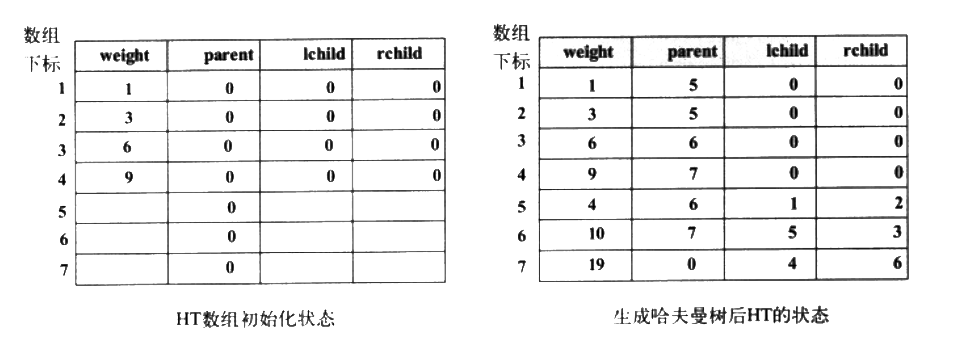展开全文哈夫曼编码 哈夫曼算法 最优二叉树
•数据结构
• 1．输出存放哈夫曼树的数组HT初态和终态； 2．输出每个字符的哈夫曼编码； 3．输入一个字符串，对字符串进行编码并输出； 4．（选作）输入一串以哈夫曼编码方式编码的二进制码，进行译码并输出。 运行截图如下，...
• ## 数据结构知识点复习（三）

千次阅读 多人点赞 2019-12-23 11:01:06
第5章 树和二叉树 5.4二叉树的性质存储结构 5.4.1二叉树的性质 有两种特殊形态的：满二叉树完全二叉树 完全二叉树的特点： （1）叶子节点只能在层次最大的两层上出现 （2）对任一节点，若其右...
• 哈夫曼树的创建及输出 #include&lt; iostream&gt; #include&lt;stdlib.h&gt; using namespace std; typedef struct { int weight; int parent, lchild, rchild; }HTNode, *HuffmanTree; void Select...
•  赫夫曼的代码 注意 我的代码都是在vs2010上运行通过的 在其他编译器上面不一定完全是好的 #define DEBU #include using namespace std; typedef struct{ unsigned int weight; ...数据结构 struct 编译器 file
• 赫夫曼（Huffman Tree），又称最优二叉树，是一类带权路径长度最短的。假设有n个权值{w1,w2,…,wn}，如果构造一棵有n个叶子节点的二叉树，而这n个叶子节点的权值是{w1,w2,…,wn}，则所构造出的带权路径长度最小...huffman
• 写出构造一棵哈夫曼树，并根据哈夫曼树求哈夫曼编码的算法。 【实验要求】 用户给定若干个整数作为待编码字符的权值，程序建立哈夫曼树并输出各字符的哈夫曼编码。 【例】设权w={5， 29， 7， 8， 14， 23， ...C语言
• #include#include#includeint m,s1,s2;typedef struct {unsigned int weight;unsigned int parent,lchild... //动态分配数组存储哈夫曼树typedef char *HuffmanCode; //动态分配数组存储哈夫曼编码表void Select(Huf...
• 实验目的：哈夫曼树的构建以及哈夫曼编码的输出 实验思想：1.先构建一个哈夫曼树  2.每个叶子节点为结点的名称  3.然后进行遍历  4.向左为0 向右为1  5.存入一个字符数组中 最后在输出 ① 头文件的构建：...数据结构
• 哈夫曼树的建立与实现.doc》由会员分享，可免费在线阅读全文，更多与《哈夫曼树的建立与实现(最终版)》相关文档资源请在帮帮文库(www.woc88.com)数亿文档库存里搜索。1、字母的总数str[j]=i+；送对应的字母到数组...
• 哈夫曼树的建立与实现.doc》由会员分享，可免费在线阅读全文，更多与《哈夫曼树的建立与实现(最终版)》相关文档资源请在帮帮文库(www.woc88.com)数亿文档库存里搜索。1、母的首地址FILE*f；文件的指针if((f=foen...
• 1.可将哈夫曼树的构建过程清楚地展现出来； 2.可通过哈夫曼树的成功构建得到哈夫曼编码； 3.可将哈夫曼树的树形结构清楚地展现出来； 此处将权值序列{8 5 29 7 8 14 23 3 11}构建成哈夫曼树； 输入各节点...数据结构 c语言
• 一、哈夫曼树 二、哈弗曼编码实现 三、总结 一、哈夫曼树 哈夫曼(Huffman)树，又称最优树，是一类带权路径长度最短的树，本文讨论最优二叉树。 下面先介绍其中的一些名词概念。 路径：从树中一个结点到另一个...数据结构 算法 c语言 c++
• ## 哈夫曼树编码-C语言

千次阅读 多人点赞 2019-11-10 17:02:45
了解二叉树的定义，理解二叉树的基本性质存储结构，掌握哈夫曼树的构造，实现哈夫曼编码与译码算法。 2.实验内容 从键盘输入一串电文字符与权值，输出对应的哈夫曼编码；从键盘输入一串二进制代码，输出对应的...数据结构 C语言 算法
• 构建HaffmanTree 上课的时候老师讲到HaffmanTree的时候，看了下书上的伪代码了解了大致的思路就开始了在自己电脑上代码实现，不过搞笑的是想着下课就给实现了... 运行结果： 对比一下书上的初态以及终态表格，没有错误
• 哈夫曼树又称作最优二叉树，是带权路径长度最小的二叉树。 一、算法步骤： 构造哈夫曼树算法的实现可以分成两大部分。 ①初始化:首先动态申请2n个单元;然后循环2n-1次，从1号单元开始，依次将1至m所有单元中的...c++ 数据结构
• Description 利用静态链表建立赫夫曼，建树过程中要求左子树权值小于右子权值，求各结点的编码。要求：叶子结点的个数n及结点值由键盘录入。本题给出程序代码,要求修改以满足测试要求. #include “stdio.h” #...
• 一、哈夫曼树的概念定义 什么是哈夫曼树？ 让我们先举一个例子。 判定树： 在很多问题的处理过程中，需要进行大量的条件判断，这些判断结构的设计直接影响着程序的执行效率。例如，编制一个程序，将百分制...
• 哈夫曼树： 所谓哈夫曼(Huffman)树就是最优二叉树，是带权路径长度WPL最小的二叉树。 哈夫曼树的构造： 根据哈夫曼树的特点：权值越大的结点离根结点越近。 具体方法：依次选择权值最小的二个结点作为左右子树构造一...二叉树 数据结构 算法
• Description 利用静态链表建立赫夫曼，建树过程中要求左子树权值小于右子权值，求各结点的编码。要求：叶子结点的个数n及结点值由键盘录入。本题给出程序代码,要求修改以满足测试要求. #include “stdio.h” #...数据结构...